﻿ On Balancing Acidic and Basic Reduction/Oxidation Reactions with a Calculator

Matthew S. Fox

## On Balancing Acidic and Basic Reduction/Oxidation Reactions with a Calculator

Matthew S. FoxDepartment of Chemistry, Cheyenne Mountain High School, Colorado Springs, Colorado, United States

### Abstract

Balancing chemical equations is a fundamental skill every chemist—from novice to professional—must have. In this report we present a calculator-based, linear algebraic method that balances even the most rigorous acidic and basic conditioned redox equations in an expeditious manner.

• Matthew S. Fox. On Balancing Acidic and Basic Reduction/Oxidation Reactions with a Calculator. World Journal of Chemical Education. Vol. 3, No. 3, 2015, pp 74-77. http://pubs.sciepub.com/wjce/3/3/4
• Fox, Matthew S.. "On Balancing Acidic and Basic Reduction/Oxidation Reactions with a Calculator." World Journal of Chemical Education 3.3 (2015): 74-77.
• Fox, M. S. (2015). On Balancing Acidic and Basic Reduction/Oxidation Reactions with a Calculator. World Journal of Chemical Education, 3(3), 74-77.
• Fox, Matthew S.. "On Balancing Acidic and Basic Reduction/Oxidation Reactions with a Calculator." World Journal of Chemical Education 3, no. 3 (2015): 74-77.

 Import into BibTeX Import into EndNote Import into RefMan Import into RefWorks

### 1. Introduction

The algebraic method for balancing chemical equations is traditionally less popular than alternative methods, as the corresponding sets of linear equations are often tedious to equate. Likewise, using algebra to balance redox equations has proven to be even more difficult, allowing other methods such as inspection or half-reactions to be more commonly taught. Balancing chemical equations with linear algebra simplifies the algebraic method; however, according to McCoy,  “Linear algebra [will] not help balance [redox equations] properly.” Many authors proceeded to disprove this proposition, [2, 3, 4] while others introduced the necessary reformulations of both chemical and redox equations to derive effective linear algebraic methods [5, 6, 7, 8, 9].

It is the purpose of this work to establish a calculator-based procedure for balancing acidic and basic conditioned redox equations. The proposed method is appropriate for undergraduate chemistry classes and perhaps Advanced Placement courses, provided scientific calculators are available. The major contribution in this report is the linear algebraic representation of the acidic and basic half-reaction procedure. This contribution introduces a new, calculator-based method, which has potential to alter how balancing redox equations is taught.

### 2. Necessary Review

This manuscript will continually refer to the following chemical equation Ac, where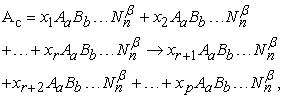where x1 through xr denote both the term and unknown coefficient for each compound reacting, xr+1 through xp the term and unknown coefficient for each compound being produced, and β the net charge associated with each compound.

The first condition for balancing any chemical equation asserts the law of conservation of mass. That is,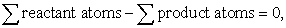where both sides of the above equation refer to the same element.  For element A in Ac specifically(1)

where a denotes the subscript for element A in each term of Ac. Reformulating eq 1 for each element in Ac yields the linear homogeneous system(2)

which is equivalent to the matrix settingIf the coefficient matrix above is denoted by A and the solution vector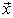, then the system follows the homogeneous equation A. [10, 11] Solving the equation foris known as computing the null space, or kernel, of the coefficient matrix A.  Thus, the kernel of a chemical equation’s coefficient matrix is also the solution to the chemical equation, provided the stoichiometric coefficients are in their simplest, whole number ratios .

The next condition asserts the net reactant charge must be equal to the net product charge.  This introduces the charge equation, denoted as β(Ac), which is nearly identical to eq 1. Formally,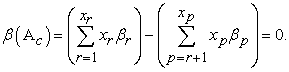(3)

If β(Ac) is appended to eq 2, then the corresponding matrix setting changes accordingly. Effectively, A gains a new row vector equivalent to the net charges associated with each compound. Thus,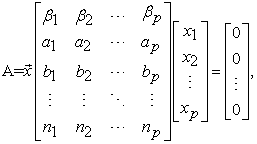(4)

which resembles the general formula to balance any redox reaction.

For example, consider the equation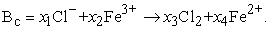Here, x1 and x2 represent the set of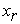, while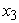andthe set of xp. Eq 3 produces β(Bc), where β(Bc) = -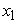+ 3+ 0- 2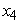= 0, with the complete homogeneous system equivalent toTherefore,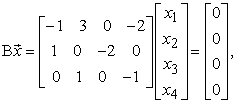which is synonymous with eq 4. (Notice how the coefficients in the above equations correspond directly to the entries in the matrix.)

The kernel of B is most commonly equated by first calculating the reduced row echelon form (rref) of matrix B. Inputting B into a scientific calculator and selecting the matrix function ‘rref’ yields(For a TI-84 calculator, input the following commands to obtain the rref: 2ND,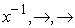, ENTER, and input the determined coefficient matrix. Once complete, input: 2ND, MODE, 2ND,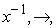ALPHA, APPS, 2ND,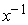, ENTER, ENTER.)

To calculate the kernel of B, take the right-most column in the rref(B) and append an additional ‘−1’ to the bottommost row. (Note that if the matrix is square, meaning the same number of rows as columns, appending the ‘1’ is not necessary) This obtainsFrom here, multiply each entry by a common factor to obtain whole number ratios and the greatest amount of positive coefficients possible. For B specifically, multiplying by a scalar of −2 yields the kernel of B, where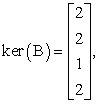or### 3. Methods

In this section we introduce the linear algebraic representation of the acidic and basic half-reaction procedure. Such representations are the main contributions of this article.

3.1. Reactions in Acidic Solutions

Remark. In this paper we denote redox equations balanced in acidic conditions as A+, where A is the coefficient matrix for the chemical equation Ac.

According to the acidic half-reaction procedure, H+ and H2O are appended to separate sides of the initial redox equation, and then balanced accordingly. The former implies the unbalanced redox equation will have two terms appended similar to H2O +…→…+ H+. The linear algebraic representation is then two column vectors appended to opposite sides of the coefficient matrix identical to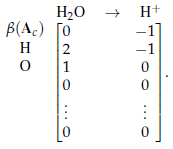(5)

Note the above row vectors will not necessarily be applied as cited above. That is, depending on how the coefficient matrix A was originally derived, β(Ac), Hydrogen, and Oxygen will not necessarily be listed in the first, second, and third rows, respectively. For sake of understanding, however, the row vectors in the examples of this paper do follow the order as shown in eq 5.

Consider the chemical equation Cc, where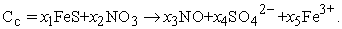The linear homogeneous system is obtained by following eq 2. Consequently,Eq 4 yields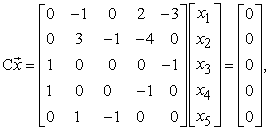which represents the matrix system for Cc. Appending eq 5 yields the systemorNotice with the addition of eq 5,gains two entries due to Cc gaining two terms: H2O and H+. Computing the null space of C+ asserts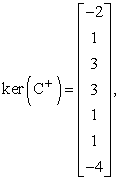or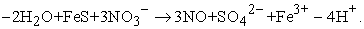To finish, the compounds with negative coefficients are transferred to the opposing side of the equation.  Ergo,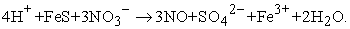3.2. Reactions in Basic Solutions

In contradistinction to eq 5, the basic half-reaction procedure implies that both OH− and H2O are added to the initial redox equation. Chemically, H2O +… →…+ OH−. Linear algebraically,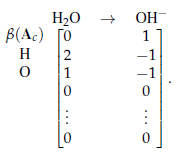(6)

(Analogous to eq 5, the implementation of eq 6 will yield A− for chemical equation Ac.) Consider the chemical equation Dc, where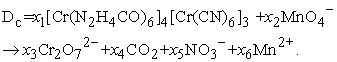Utilizing eq 2, the linear homogeneous system is established. That is,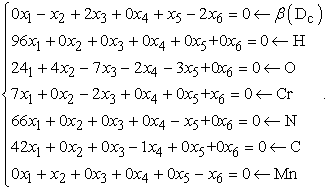Thus,Affixing eq 6 yields the system, whereThe rref() produces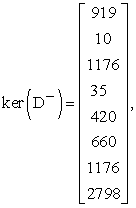or### 4. The Mathematical Procedure

The following list enumerates the procedure to balance acidic or basic redox equations in an efficient, computational process.

1. Be sure the equation is in its respective net ionic representation.

2. From the information given in the redox reaction, produce homogeneous equations for each element.

3. Establish the homogeneous charge equation from the unique charges associated with each compound.

4. Transform the equations into a matrix system, where each row represents each element and each column represents each term in the system’s coefficient matrix.

5. Depending on the information in the redox equation and whether the equation is to be balanced in either acidic or basic conditions, append either eq 5 or eq 6, respectively.

6. Equate the kernel of the coefficient matrix developed in the preceding step by utilizing a calculator’s reduced row echelon form (rref) matrix function.

7. Check that the kernel of A has entries in the lowest possible whole number ratios with the greatest number of positive coefficients possible.

8. Insert the kernel into the chemical equation and check that the equation is balanced. If negative coefficients appear in the final equation, transfer these coefficients and their corresponding compounds to the opposing side of the equation.

4.1. Limitations

The rref(A) is only effective if the order of A is, where m = n, or m < n by only a difference of 1. Fortunately, most redox equations follow these parameters, especially after eq 5 or eq 6 is appended. In principle, however, if an unbalanced redox equation has a number of different elements > the number of unknown coefficients (m > n), the linear algebraic method cannot be used.

### 5. Conclusion

The linear algebraic representation of the acidic and basic half-reaction procedure was introduced in this paper. The representation established a calculator-based method to balance redox equations in either acidic or basic conditions. The efficiency and intuitiveness associated with the proposed method allows students to balance rigorous redox reactions in an expeditious fashion, while not undermining the essential laws governing balanced reactions.

A potential criticism regarding the proposed method is intuition on the linear algebra used to produce the chemical result. Linear algebra is a complex subset of mathematics traditionally offered to sophomores and juniors in college. It is for this reason that the method is not rigorously proven to yield the chemical solution. Albeit a proof does exist, it is unnecessary to show as the procedure is calculator based; and as such neither the student nor the teacher is responsible for linear algebraic interpretation—only chemical.

### Competing Interests

The author declares no competing financial interest.

### Acknowledgements

This manuscript was supported in part by Janie Mueller, Zack Casias, Dave Hale, Ben Jakeman, and Logan Patterson.

### References

  McCoy, B. Application of Linear Algebra: Balancing Chemical Equations. The University of North Carolina t Chapel Hill. http://www.unc.edu/_marzuola/Math547 S13/Math547S13 Projects/B McCoy Section001 BalancingChemicalEquations.pdf (accessed August 2014).In article  Kolb, B. More on Balancing Redox Equations. J. hem. Educ. 1979, 56, 181.In article  Carrano, S.A. Balancing an Atypical Redox Equation. J. Chem. Educ. 1978, 55, 382.In article View Article  Olson, J.A. An Analysis of the Algebraic Method for Balancing Chemical Reactions. J. Chem. Educ. 1997, 74 (5), 538-542.In article View Article  Kennedy, J.H. Balancing Chemical Equations with a Calculator. J. Chem. Educ. 1982, 59, 523.In article View Article  Blakeley, G.R. Chemical Equation Balancing: A General Method which is Quick, Simple, and has Unexpected Applications. J. Chem. Educ. 1982, 59, 728.In article View Article  Porter, S.K. How Should Equation Balancing be Taught?. J. Chem. Educ. 1985, 62, 507.In article View Article  Alberty, R.A. Chemical Equations are Actually Matrix Equations. J. Chem. Educ. 1991, 68, 984.In article View Article  Alberty, R.A. Conversion of Chemical Equations to Biochemical Equations. J. Chem. Educ. 1992, 69 (6), 493.In article View Article  Strang, G. Linear Algebra and Its Applications, 4th ed.; Academic Press, INC.: London, 2006; pp 73.In article  Risteski, I.B. A New Singular Method for Balancing Chemical Equations and Their Stability. J. of the Chinese Chem. Society. 2009, 56, 68.In article